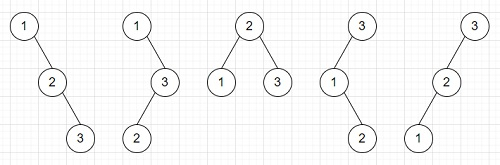# Program to count number of BST with n nodes in Python

Suppose we have n different nodes. All are distinct. We have to find how many number of ways we can arrange them to form Binary search tree. As we know for binary search trees, the left subtree always hold smaller values and right subtrees hold the greater values.

To solve this, we shall find the Catalan number. The Catalan number C(n) represents the binary search trees with n different keys. The formula is like

$$C(n)=\frac{(2n)!}{(n+1)!\times n!}$$

So, if the input is like n = 3, then the output will be 5 becauseTo solve this, we will follow these steps −

• Define a function ncr() . This will take n, r
• res := 1
• if r > n - r, then
• r := n - r
• for i in range 0 to r - 1, do
• res := res *(n - i)
• res := floor of (res/(i + 1))
• return res
• From the main method, do the following
• c := ncr(2 * n, n)
• return floor of c /(n + 1)

## Example

Let us see the following implementation to get better understanding −

from math import factorial

def ncr(n, r):
res = 1
if r > n - r:
r = n - r

for i in range(r):
res *= (n - i)
res //= (i + 1)

return res

def solve(n):
c = ncr(2 * n, n)
return c // (n + 1)

n = 3
print(solve(n))

## Input

3


## Output

5